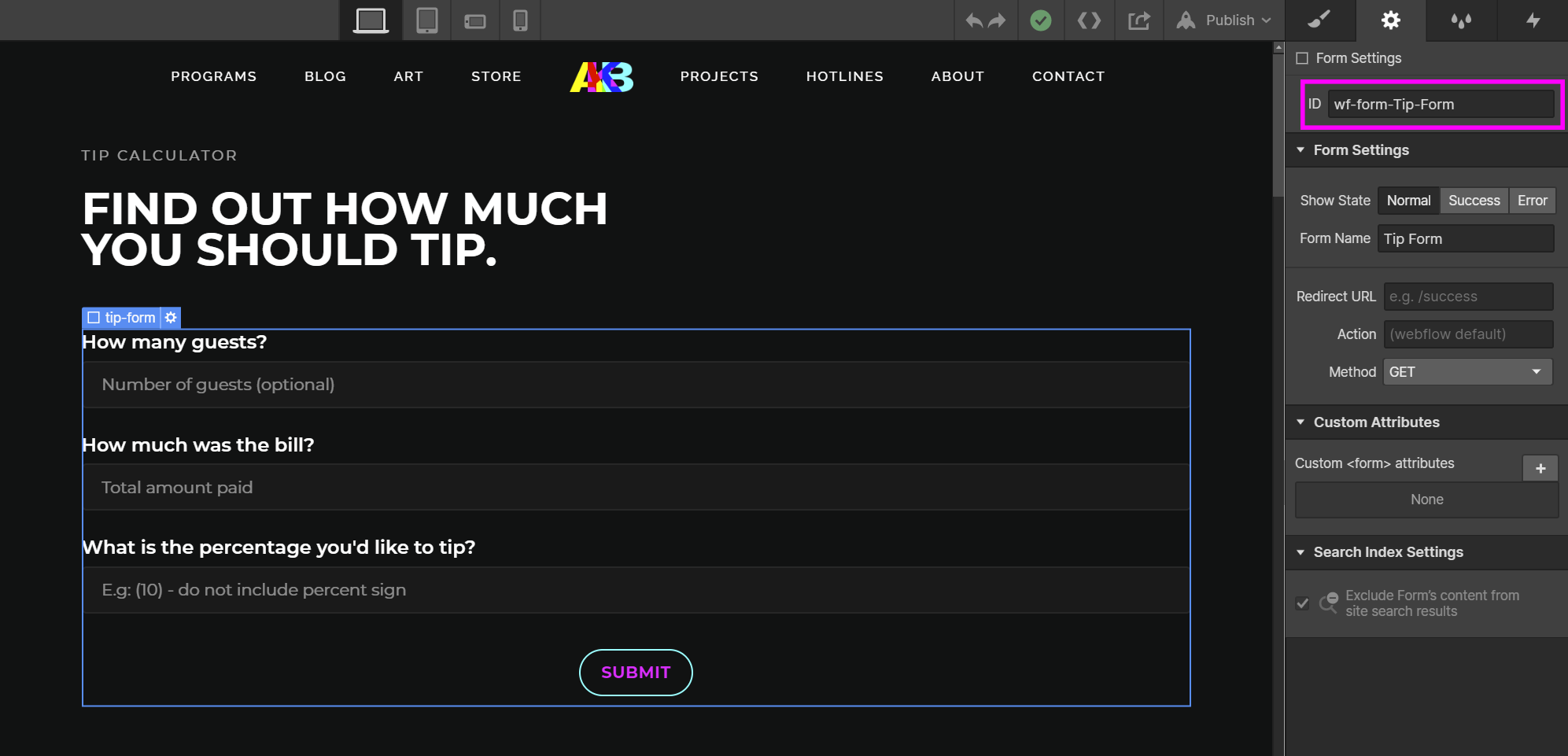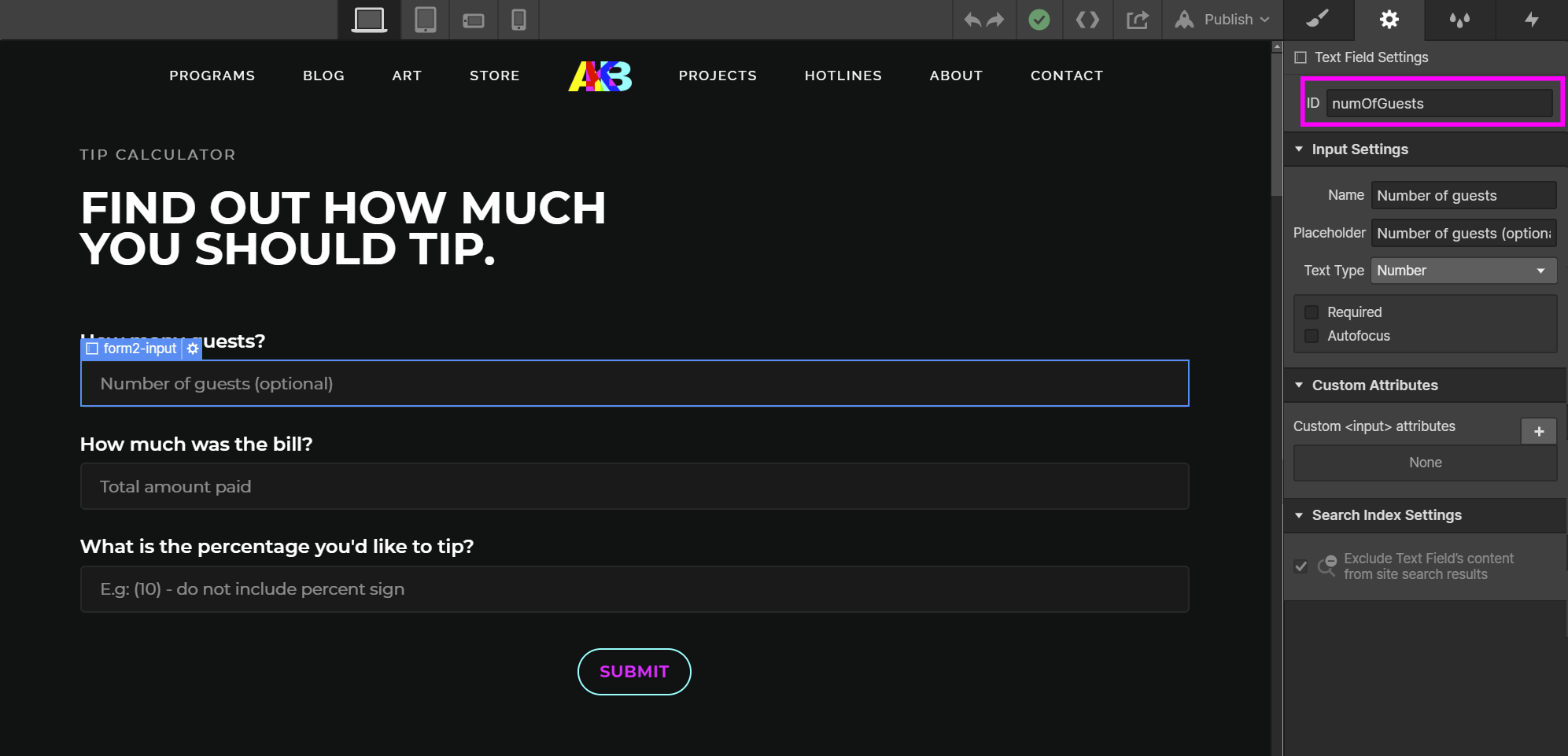tip calculator

## find out how much you should tip.Oops! Something went wrong while submitting the form. That's on us. Try refreshing the page.
1. Create the form using Webflow's built-in form block.
Make sure to set the ID of the heading, the form, its text fields and its success message (it will be necessary later)Click the element you want to set an ID for, navigate to its settings and enter a value in for its ID. I showed some examples above, but I also had to set IDs for the heading and the success message to manipulate them in the script.
2. Calculate the tip amount based on the percentage entered in the form.
The IDs are used in this step. The form ID is used to create the variable "tipForm" which will be used to add an event listener to the form.
``````<script type="text/javascript">

let tipForm = document.getElementById("wf-form-Tip-Form");

// initializing variables
let tipAmount;
let tipAmountPerGuest;

function tipCalculator(numOfGuests, totalAmountPaid, percentOfTip){

/*** CALCULATE PERCENTAGE
* Multiply the number by the percent (e.g. 87 * 68 = 5916)
* Divide the answer by 100 (Move decimal point two places to the left)
(e.g. 5916/100 = 59.16)
*/

// handling edge cases
if (numOfGuests < 1) {
numOfGuests = 1;
}

// using the percentage formula to calculate the tip based on the tip percent entered
tipAmount = (totalAmountPaid * percentOfTip) / 100;
tipAmount = Math.round(100*tipAmount)/100;

// calculate the amount each guest should tip
tipAmountPerGuest = tipAmount / numOfGuests;
tipAmountPerGuest = Math.round(100*tipAmountPerGuest)/100;
}
``````
4. Create a function to display the tip information in the success message of the form.
The IDs are used in this step. The form heading ID is used to set the heading to "Your tip information: " and the success message ID is used to set the success message to the tip information.
``````    function display(){

let tipInformation = ["The total amount you should tip is: \$" + tipAmount,
"Each guest should tip: \$" + tipAmountPerGuest].join("\n");

// copying new title to the form heading

// styling the success msg using pre-defined classes
let successMsg = document.getElementById("success-msg");
successMsg.style.whiteSpace = "pre";

// copying tio information to the success msg
successMsg.innerText = tipInformation;
successMsg.textContent = tipInformation;
}
``````
5. Create a wrapper function to call the display function.
The IDs are used in this step. The field IDs are used to get the value of the data entered in the tipPercent, numOfGuests and totalAmountPaid fields to pass in the tipCalculator function. The tipForm variable created in Step 2 is used to add an event listener that executes the script when the form is submitted.
``````    function startDisplay(){
let percentOfTipData = document.getElementById("tipPercent").value;
let numOfGuestsData = document.getElementById("numOfGuests").value;
let totalAmountPaidData = document.getElementById("totalAmountPaid").value;

// calculate tip and tip per guest
tipCalculator(numOfGuestsData, totalAmountPaidData, percentOfTipData);

// display the tip on the web page
display();
}
</script>
``````
The entire script should look like:
The IDs are used in this step. The field IDs are used to get the value of the data entered in the tipPercent, numOfGuests and totalAmountPaid fields to pass in the tipCalculator function. The tipForm variable created in Step 2 is used to add an event listener that executes the script when the form is submitted.
``````<script type="text/javascript">

let tipForm = document.getElementById("wf-form-Tip-Form");

// initializing variables
let tipAmount;
let tipAmountPerGuest;

function tipCalculator(numOfGuests, totalAmountPaid, percentOfTip){

/*** CALCULATE PERCENTAGE
* Multiply the number by the percent (e.g. 87 * 68 = 5916)
* Divide the answer by 100 (Move decimal point two places to the left) (e.g. 5916/100 = 59.16)
*/

// handling edge cases
if (numOfGuests < 1) {
numOfGuests = 1;
}

// using the percentage formula to calculate the tip based on the tip percent entered
tipAmount = (totalAmountPaid * percentOfTip) / 100;
tipAmount = Math.round(100*tipAmount)/100;

// calculate the amount each guest should tip
tipAmountPerGuest = tipAmount / numOfGuests;
tipAmountPerGuest = Math.round(100*tipAmountPerGuest)/100;
}

function display(){

let tipInformation = ["The total amount you should tip is: \$" + tipAmount,
"Each guest should tip: \$" + tipAmountPerGuest].join("\n");

// copying new title to the form heading

// styling the success msg using pre-defined classes
let successMsg = document.getElementById("success-msg");
successMsg.style.whiteSpace = "pre";

// copying tio information to the success msg
successMsg.innerText = tipInformation;
successMsg.textContent = tipInformation;
}

function startDisplay(){
let percentOfTipData = document.getElementById("tipPercent").value;
let numOfGuestsData = document.getElementById("numOfGuests").value;
let totalAmountPaidData = document.getElementById("totalAmountPaid").value;

// calculate tip and tip per guest
tipCalculator(numOfGuestsData, totalAmountPaidData, percentOfTipData);

// display the tip on the web page
display();
}